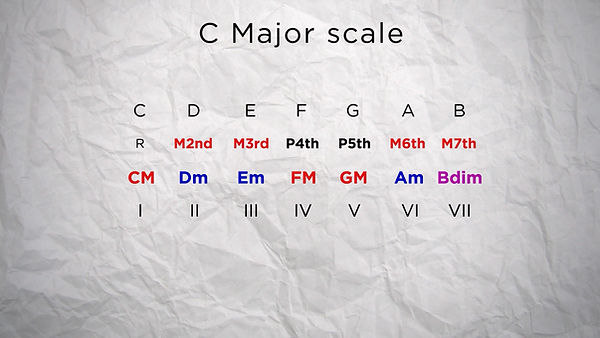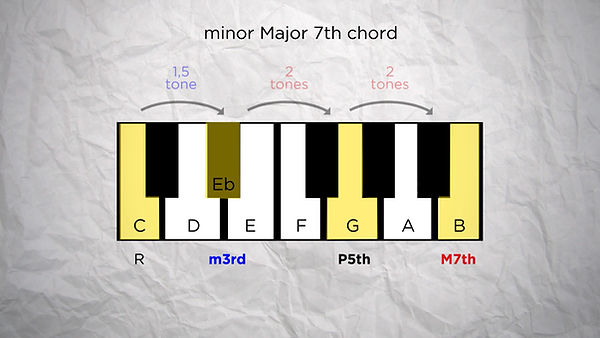top of page# 06. 7th chords

By stacking thirds on top of each other, or taking one note every 2, you can create chords. But you don't have to stop at a triad, with 3 notes, you can add more.

If you add 1 extra note, you add the 7th, so that's a 7th chord.
If you add another extra note, you add a 9th, so that's a 9th chord.
Another extra note and you're adding a 11th, so that's called a 11th chord.
Another extra note and you're adding a 13th, so that's called a 13th chord. You get it.

If I try to make a 13th chord from C in the C major scale, that makes C E G B D F A. So technically this... is a 13th chord. Yeah!Again, the nature of these extended chords will depend on the intervals between each of their notes.
So let define all these intervals once and for all.

INTERVALS

Every interval is define by the distance between a note of the chord and the root note.
Some intervals will be called perfect. If they're extended by a semi tone they're called augmented, and if they're shortened by a semi tone they're called diminished.

Then some intervals are either major or minor, with a semitone between the two states. If a major interval is extended by 1 semi tone it becomes augmented, and if a minor interval is shortened by 1 semi tone it becomes diminished. But these are more rare instances.To know which intervals are perfect or major/minor, just remember the C major scale, as everything seems to be built around that. That's all the white keys on the keyboard.

In the C major scale the fourth and the fifth are "perfect intervals" and the second, third, sixth and seventh are all major intervals.
From there it gives you all the reference intervals you need, to know your sixth or seventh is minor or major.
From there, it gives you all the reference intervals you need to know if your 6th or 7th is minor or major.For example, if you have 4 tones between two notes, that's like the interval between a C and a G# or Ab, so that's an interval of either an augmented 5th or a minor 6th.For the 7th, it is a M7th if the note is 5,5 tones above the root note. That a semi - tone below its octave. Or if it is 2 tones above the perfect 5th. It becomes a m7th F it's a whole tone below the root note, or 1,5 above the perfect fifth.

We can also observe that the major chords in this scales are built upon the perfect intervals, and the minor (or diminished) chords are built upon the non-perfect intervals.This figure can come in very handy as a reference, and should definitely be on a cheat sheet if you need one.

So now that we know how to build a 7th chord - by adding a 7th on top of a triad - and we know how we can identify different intervals, let's see the most used 7th chords.

7th CHORDS:

A major triad with a minor 7th (which would be a minor 3rd above the fifth) is probably the most common 7th chord and is called a "dominant 7th chord", because it is often used on the Vth degree, which is the dominant degree.
For a C chord that will make C E G Bb.
It would be noted C7If we have a major triad with a major 7th, that would make a major 7th chord.
So for C that would be C E G B and would be noted CM7Then if we have a minor triad with a minor 7th, that makes a minor 7th chord.
So for a C chord that would be C Eb G Bb, that would be noted Cm7You can also have a minor triad with a major 7th, that would be a minor major 7th chord.
That would make for instance C Eb G B that is noted CmM7. This one is a bit more rare.Then we can have an diminished triad with a minor 7th, that'd be called a half-diminished 7th chord,
So for example C Eb Gb Bb that would be noted Cm7b5 or C⍉ (with a crossed circle, that a half-diminished 7th chord)And to have a fully diminished 7th chord, it would be a diminished triad with a diminished 7th.
So for C it would be C Eb Gb Bbb.
I like this one because it's a bit confusing.
Even though the B technically becomes the same note than a A, it still serves the function of a 7th, as we stack up minor third on top of each other to make this one. But A would be the 6th, so we keep the name B for that note to show that it's considered a 7th. But it's double flat, it's diminished.
So the C diminished 7th chord would be noted Cdim7
This one is very dissonant as it has two intervals of diminished fifths in it, and I like it a lot!Now with an augmented triad, we can have a minor 7th. That would be called an augmented 7th chord.
So on a C chord, that would be C E G# Bb

This one is sort of a break in the rule, as it doesn't have an interval of a 3rd between its fifth and its 7th. This chord is also used a lot on chords of Vth degree, and is also known as dominant augmented seventh chord.
That woulbe be noted C+7.With augmented triad, we can have a major 7th. That would be called a augmented major 7th chord.
So on a C chord that would be C E G# B and that would be noted CM7#5 or C+M7Now that we know what the different 7th chords look like, we can identify each 7th chord of our C major scale.

7th CHORDS IN A SCALE:

If we take each note of the scale and build their 7th chords - so remember, you start with the root note then take one note every two - and see the interval that compose them, we find that the C chord, the Ist degree, is a CM7.
The II degree is a Dm7
The III degree is a Em7
The IV degree is a FM7
The V degree is a G7
The VI is a Am7
And the VII is a B⍉This gives you the states of all the 7th chords in a major scale.
Because all major scales have a relative minor scale that use the exact same notes, you can have the 7th chords of a minor scale by simply starting from the VIth degree, because that's were the relative minor scale begins. So these are the 7th chords of a minor scale.You can add other notes on top of that to create 9th, 11th or 13h chords but that may already be a lot of information, and I would like to talk a bit about how to actually use these chords to create our chord progressions first.bottom of page